Other Parts Discussed in Post: TLV333, TLV2333, TLV4333

Many sensors produce low-level DC outputs that require a high input-impedance amplification stage to increase the signal amplitude. Sensors used in personal and portable electronics require operational amplifier (op amp) circuits that provide high input impedance and DC precision, while also being low power and cost-effective.

In this post, I’ll explain how to design a few cost-optimized low-power DC-accurate circuits using TLVx333 op amps in different circuit configurations. These devices provide high levels of DC accuracy with maximum input offset voltages (VOS) less than 15µV, and a typical VOS drift of 0.02µV/°C. The 0.1Hz to 10Hz low-frequency noise specification is only 1.1µVpp and the 0.01 – 1Hz noise specification is only 0.3 µVpp. Table 1 shows the key performance metrics for TLVx333 family.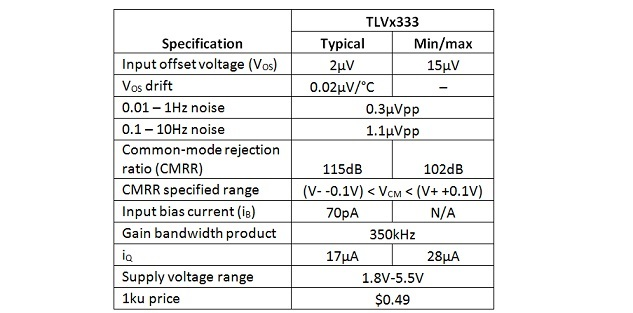Table 1: Key specifications for the TLV333

Single-ended sensors can interface with standard noninverting amplifier circuits, as shown in Figure 1. The transfer function is shown in Equation 1. Noninverting op amp circuit-offset errors are dominated by the input offset voltage (VOS) and the VOS temperature drift of the op amp. Additional offset errors come from the CMRR and the input bias current of the op amp. The tolerance and temperature coefficient of the resistors in the feedback network set the gain error and gain-error drift. The circuit shown in Figure 1 is configured for a gain of 500V/V, and the closed-loop bandwidth is 1.14kHz.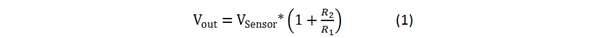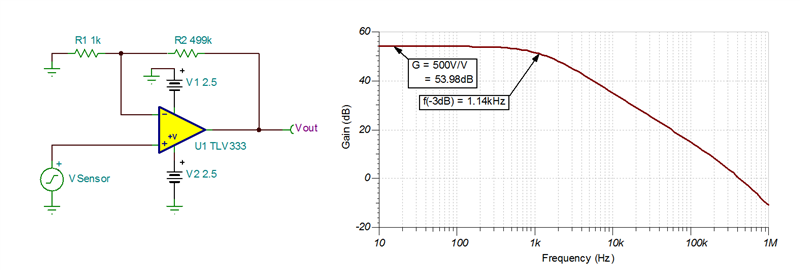Figure 1: TLV333 used in a noninverting amplifier configuration

Sensors with differential outputs such as bridge sensors and strain gauges require a circuit with differential inputs. One of the simplest options to interface with a differential sensor is the four-resistor difference amplifier circuit shown in Figure 2. If R1 is set equal to R3 and R2 is set equal to R4, then the transfer function simplifies to Equation 2. The tolerance of the resistors in the difference amplifier will directly affect the CMRR of the circuit. Selecting 0.1% resistors achieves at least 54dB of CMRR, while 0.01% resistors achieve at least 74dB. Note that discrete difference amplifier designs will typically not match the performance of integrated solutions, but they often offer advantages in flexibility and cost. The circuit in Figure 2 is configured for a gain of 499V/V, with a closed-loop bandwidth of 1.16kHz.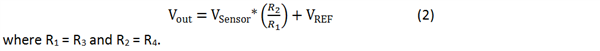Figure 2: TLV333 used in a difference amplifier configuration

High-impedance sensors with differential outputs often require circuits with input impedances >1MΩ. Achieving input impedances >1MΩ is often not practically possible using a discrete difference amplifier topology. Large resistors will increase the DC errors from input bias current, increase circuit intrinsic noise, increase susceptibility to extrinsic noise and will likely require stability compensation.

Figure 3 shows a discrete two-op-amp instrumentation amplifier (INA) using a dual-channel TLV2333. The two-op-amp INA presents a high-impedance differential input to the sensor while only requiring two op amps and five precision resistors. Assuming that R1 is set equal to R3 and R2 is set equal to R4, Equation 3 shows the transfer function. The circuit in Figure 3 is configured for a gain of 500V/V, with a closed-loop bandwidth of 1.02kHz.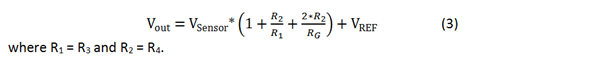You can also construct a discrete three-op-amp INA using a dual-channel op amp, a single-channel op amp and seven precision resistors. Equation 4 shows the transfer function for the three-op-amp INA. INA designs often require a buffer for a high-impedance reference or an op amp used as an integrator to high-pass filter the input signal. Figure 4 shows a TLV4333 used to create a three-op-amp INA with a reference buffer. The circuit in Figure 4 is configured for a gain of 500V/V and has a closed-loop bandwidth of 1.16kHz.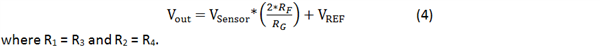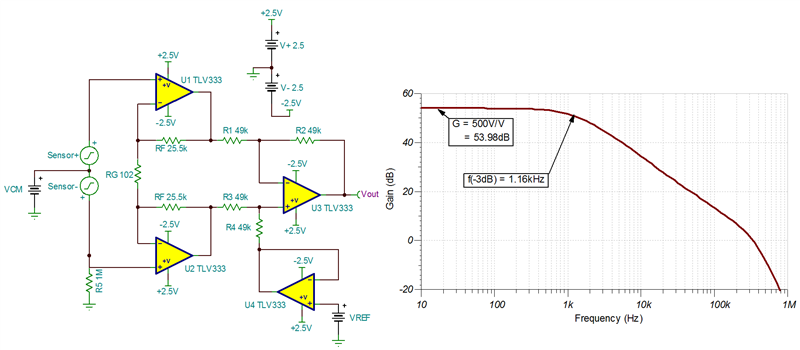You can use the TLVx333 family of devices in several ways to create DC-accurate circuits that are ideal for cost-optimized precision-sensor acquisition and precision-instrumentation applications.